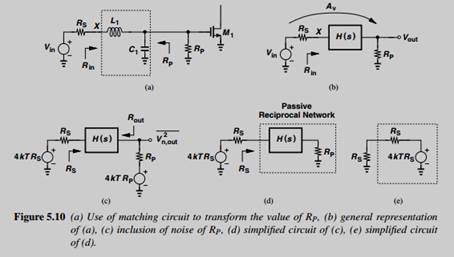Create an Account

Home / Questions / Repeat Example 5.5 by solving the specific network shown in Fig 5 10 a Example 5 5 A st...

Repeat Example 5.5 by solving the specific network shown in Fig 5 10 a Example 5 5 A student decides to defy the above observation by choosing a large RP and transforming its value down to

Repeat Example 5.5 by solving the specific network shown in Fig. 5.10(a).

Example 5.5

A student decides to defy the above observation by choosing a large RP and transforming its value down to RS. The resulting circuit is shown in Fig. 5.10(a), where C1 represents the input capacitance of M1. (The input resistance of M1 is neglected.) Can this topology achieve a noise figure less than 3 dB?Jul 31 2020 View more View LessSubscribe To Get Solution